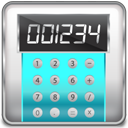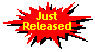#bdcalc - a calculator for large natural numbers

bdcalc is a command-line calculator and mini-programming language that works with very large unsigned integers, the natural numbers ℕ = { 0,1,2,…} used to carry out computations in cryptography. The numbers can theoretically be of unlimited size.

bdcalc has built-in functions (see below) to carry out number theoretical computations such as modular exponentiation and modular inversion, as used in the RSA and Diffie-Hellman algorithms. You can generate random prime numbers of a given bit length and test for primality.

bdcalc provides a mini-programming environment with assignments to stored variables, conditional statements (if-then-else) and control loops (for, while, repeat). See the documentation for more details.

Affiliate disclosure: we get a small commission for purchases made through the above links

You can use bdcalc in interactive mode, typing commands on the console until you type `quit` to exit. Alternatively you can read input directly from a script file using the `-file` option; or you can enter your input in one line on the command line, perhaps as part of a batch file.2016-06-17: Released new version 2.2 - see What's new

## Example session

bdcalc lets you assign numbers to variables, do arithmetic and call functions in the way you would expect.

```> a=99
99
> b=a*11
1089
> ? a+b
1188
> println("a =",a,"b =",b)
a = 99 b = 1089
> p=genprime(128)
187188849449410847765185120249130623559
> !p
0x8cd342d39544246b9743c7b68d5da247
> bitlen(p)
128
> ??isprime(p)
true
> x=1;y=2;z=x+y;?z
3
> for i in (1..15) do print(prime(i), " ") done; println("")
2 3 5 7 11 13 17 19 23 29 31 37 41 43 47
```

## Built-in Functions

Function Returns Remarks
`bitlen(X)` the bit length l of the integer `X` 2l−1 ≤ x < 2l
`bytelen(X)` the length L of integer `X` in bytes
`cbrt(X)` the truncated integer cube root of `X` 3√{x} ⎦
`compl(X,n)` the bitwise NOT of the rightmost `n` bits of `X`
`fillbytes(n,b)` an integer of `n` bytes each set to `b mod 256`
`gcd(X,Y,Z,...)` the greatest common divisor of `X,Y,Z,...`
`genprime(n)` a random prime p of length exactly `n` bits 2n−1 ≤ p < 2n
`getbit(X,n)` value 0 or 1 of bit `n` of `X` =(bl−1…bn…b1b0)
`getbyte(X,n)` value of byte `n` of `X`
`iif(B,X,Y)` `X` if `B` is true, otherwise `Y` [Note 1]
`isprime(X)` `true` if `X` is prime, otherwise `false` [Note 2]
`jacobi(X,M)` the Jacobi symbol `(X | M)` in {0,1,2},  2 ⇒ −1 [Note 3]
`max(X,Y,Z,...)` the maximum of `X,Y,Z,...`
`min(X,Y,Z,...)` the minimum of `X,Y,Z,...`
`modexp(X,Y,M)` `X` raised to the power `Y` modulo `M` xy mod m
`modinv(X,M)` the inverse of `X` modulo `M` x−1 mod m
`modmul(X,Y,M)` `X` multiplied by `Y` modulo `M` xy mod m
`modpowof2(X,n)` `X` with all bits cleared at positions ≥ `n`x mod 2n
`pow(X,n)` `X` raised to the power `n` xn
`prime(n)` the `n`-th prime number 1 ≤ n ≤ 104
`randbits(n)` a random integer r of length at most `n` bits 0 ≤ r < 2n
`random(M)` an integer r generated at random from [0, M-1] 0 ≤ r < M
`revbytes(X,[n])` `X` as an `n`-byte integer with its byte order reversed
`setbit(X,n,b)` `X` =(bl−1…bn…b1b0) with bit `n` set to value `b` b ∈ {0,1}
`setbyte(X,n,b)` `X` with byte `n` set to value `b mod 256`
`sha1(X,n)` the SHA-1 hash of the rightmost `n` bits of `X` [Note 4]
`sha256(X,n)` the SHA-256 hash of the rightmost `n` bits of `X` [Note 4]
`sqrt(X)` the truncated integer square root of `X` ⎣√x ⎦
`square(X)` the square of `X` x2

new in version 2.2

For notes and examples of use, see the manual.

## Displaying numbers in decimal and hexadecimal

To enter a number in hexadecimal, prefix it with "0x". It will display automatically in decimal. To display a number in hexadecimal, print with the "!" command.

```> 0xdeadbeefcafebabebeddeddecadedeed
295990755076957304711832595911747231469
> ! 295990755076957304711832595911747231469
```

## Example scripts

`qr_find.bdscr`
How to find a quadratic residue by exhaustive search (source)
`discretelog.bdscr`
Compute discrete logarithm (source)
`primes1000.bdscr`
Write out the first 1000 primes (source)
`rsa_make.bdscr`
Make an RSA key pair and test it (source)
`rsa_make_exact.bdscr`
Make an RSA key pair of exact bit length (source)
`rsa_quint.bdscr`
Perform RSA calculation with private key in CRT quintuple form (source)
`rsacrack.bdscr`
Crack RSA if used incorrectly to three recipients (source)
`rDSA.bdscr`
Example of the rDSA algorithm from ANSI X9.31 (source)
`dsa_test.bdscr`
Example of DSA from Appendix 5 FIPS PUB 186-2 (source)
`rsa_quint.bdscr`
Perform RSA calculation with private key in CRT quintuple form (source)
`dh_gen.bdscr`
Generate domain parameters for Diffie-Hellman (source)
`dh_keyexch.bdscr`
Perform Diffie-Hellman key exchange using parameters generated above (source)
`poly1305.bdscr`
Poly1305 Example and Test Vector (source)

## Documentation

bdcalc manual in PDF

bdcalc manual in HTML

Unzip the zip file and run the `setup.exe` program inside it, or download the exe program directly and run it. The latest version is 2.2.1.0 released 26 July 2016.

Windows binary: bdcalc is a simple stand-alone Windows EXE file just 135 kB in size. If you just want the plain `bdcalc.exe` file to install yourself, plus the help and sample scripts, download bdcalc-files.zip (381 kB) `[md5=1d594263ec22d75f6a72d6ea1591f764]`.

Linux x86 binary: Compiled with static library included. bdcalc-linux.x86.zip (529 kB) `[md5=29dd079e2c5dc1b60c12893de65a3c40]` (install notes)

Mac OSX binary: bdcalc-osx.zip (340 kB) `[md5=c03926a7936c7970bef85dedf5c40e67]` (install notes)

Please note it is a breach of copyright to put a copy of these installation files on another server or to distribute them in any manner except by providing a link to this page.

## What's new in v2.2?

New version 2.2 released 17 June 2016. This fixes up a few annoyances we had with print and help and adds a couple of new functions.

• Changed behaviour of `print` and `println` to insert spaces between arguments.
• Added `setsep` command to change print separation character.
```> println(10,20,30)   # New behaviour in v2.2
10 20 30
> setsep ''
> println(10,20,30)   # Old behaviour
102030
> setsep ', '
> println(10,20,30)   # Fancy stuff
10, 20, 30
```
• Removed requirement to put quotes around arguments for `help`.
```> help modexp   # Old behaviour - should be ``help 'modexp'``
ERROR: syntax error (incomplete line)

> help modexp   # New behaviour - no quotes required
`modexp(X,Y,M)` returns X raised to the power Y modulo M
```
• Changed error warning from `ERROR:` to `BDCALC ERROR:`. We found ourselves opening a bdcalc interactive console, leaving it for a while, and then wondering why we couldn't type a normal command. Having the word "BDCALC" there gives you a clue.
```C:\> bdcalc
BDCALC: v2.2.0 <www.di-mgt.com.au/bdcalc.html>
Enter commands: type `quit` to finish or `help` for help.
> a = 123456789
123456789
> # leave this console open and forget...
> dir file
BDCALC ERROR: syntax error at symbol 'file'
> dir file
BDCALC ERROR: syntax error at symbol 'file'
> # WTF why isn't the dir command working?
```
• Added new functions `sha256` and `fillbytes`. Here's a cute example to compute the SHA-256 digest of one million repetitions of the character "a" (ASCII 0x61). Ref: Test vectors for SHA-1, SHA-2 and SHA-3. But don't try and print out b - it's a huge number with 2.4 million digits! Make sure you use the `verbose off` statement to avoid the default behaviour of displaying the last result.
```> verbose off
> b = fillbytes(1000000, 0x61)
> ? bytelen(b)
1000000
> !sha256(b, bytelen(b)*8)
0xcdc76e5c9914fb9281a1c7e284d73e67f1809a48a497200e046d39ccc7112cd0

> # wow, what does b look like?
> ? b     # ... OH NO! ...
```
• Made second argument optional for `revbytes(X,n)`. If the n argument is omitted it defaults to `bytelen(X)`. Be careful, there are corner cases where this may not be what you want.
```> showhex on   # New feature in v2.2 to display last result in hex not decimal
• Added `showhex` statement to switch between hexadecimal and decimal displays of the result in interactive mode. See example above.